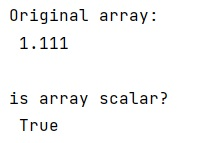# Why are 0d arrays in Numpy not considered scalar?

Learn, why are 0d arrays in NumPy not considered scalar in Python?
Submitted by Pranit Sharma, on January 13, 2023

NumPy is an abbreviated form of Numerical Python. It is used for different types of scientific operations in python. Numpy is a vast library in python which is used for almost every kind of scientific or mathematical operation. It is itself an array which is a collection of various methods and functions for processing the arrays.

A 0d array is scalar. scalars in Numpy should have the .shape and .ndim attributes, so at least the ufuncs do not have to do explicit type checking on its input for the 21 possible scalar types in Numpy. However, when we talk about the 0-dimensional array it means its ndim is 0.

Actually , the Numpy developer's solution is to inherit from both ndarray and Python scalars for its own scalar type, so that all scalars also have .shape, .ndim, .T, etc. The 1x1 matrix will still be there, but its use will be discouraged if we know we would be dealing with a scalar.

Let us understand with the help of an example,

## Python code to demonstrate the example 'why are 0d arrays in Numpy not considered scalar'

```# Import numpy
import numpy as np

# Creating a numpy array
arr = np.float64(1.111)

# Display original array
print("Original array:\n",arr,"\n")

# check for scalar
sc = np.isscalar(arr)

print("is array scalar?\n",sc)
```

Output:What's New (MCQs)

Top Interview Coding Problems/Challenges!

IncludeHelp's Blogs

Languages: » C » C++ » C++ STL » Java » Data Structure » C#.Net » Android » Kotlin » SQL
Web Technologies: » PHP » Python » JavaScript » CSS » Ajax » Node.js » Web programming/HTML
Solved programs: » C » C++ » DS » Java » C#
Aptitude que. & ans.: » C » C++ » Java » DBMS
Interview que. & ans.: » C » Embedded C » Java » SEO » HR
CS Subjects: » CS Basics » O.S. » Networks » DBMS » Embedded Systems » Cloud Computing
» Machine learning » CS Organizations » Linux » DOS
More: » Articles » Puzzles » News/Updates